Courses

# The Three Moment Equations-I (Part - 1) Civil Engineering (CE) Notes | EduRev

## Civil Engineering (CE) : The Three Moment Equations-I (Part - 1) Civil Engineering (CE) Notes | EduRev

The document The Three Moment Equations-I (Part - 1) Civil Engineering (CE) Notes | EduRev is a part of the Civil Engineering (CE) Course Structural Analysis.
All you need of Civil Engineering (CE) at this link: Civil Engineering (CE)

Instructional Objectives

After reading this chapter the student will be able to

1. Derive three-moment equations for a continuous beam with unyielding supports.
2. Write compatibility equations of a continuous beam in terms of three moments.
3. Compute reactions in statically indeterminate beams using three-moment equations.
4. Analyse continuous beams having different moments of inertia in different spans using three-moment equations.

Introduction

Beams that have more than one span are defined as continuous beams. Continuous beams are very common in bridge and building structures. Hence, one needs to analyze continuous beams subjected to transverse loads and support settlements quite often in design. When beam is continuous over many supports and moment of inertia of different spans is different, the force method of analysis becomes quite cumbersome if vertical components of reactions are taken as redundant reactions. However, the force method of analysis could be further simplified for this particular case (continuous beam) by choosing the unknown bending moments at the supports as unknowns. One compatibility equation is written at each intermediate support of a continuous beam in terms of the loads on the adjacent span and bending moment at left, center (the support where the compatibility equation is written) and rigid supports. Two consecutive spans of the continuous beam are considered at one time. Since the compatibility equation is written in terms of three moments, it is known as the equation of three moments. In this manner, each span is treated individually as a simply supported beam with external loads and two end support moments. For each intermediate support, one compatibility equation is written in terms of three moments. Thus, we get as many equations as there are unknowns. Each equation will have only three unknowns. It may be noted that, Clapeyron first proposed this method in 1857. In this lesson, three moment equations are derived for unyielding supports and in the next lesson the three moment equations are modified to consider support moments.

Three-moment equation

A continuous beam is shown in Fig.12.1a. Since, three moment equation relates moments at three successive supports to applied loading on adjacent spans, consider two adjacent spans of a continuous beam as shown in Fig.12.1b. ML, MC and MR respectively denote support moments at left, center and right supports. The moments are taken to be positive when they cause tension at bottom fibers. The moment of inertia is taken to be different for different spans. In the present case IL, and IR denote respectively moment of inertia of; left and right support and  IL, and IR are the left and right span respectively. It is assumed that supports are unyielding. The yielding of supports could be easily incorporated in three-moment equation, which will be discussed in the next lesson. Now it is required to derive a relation between ML, MC and MR . This relationship is derived from the fact that the tangent to the elastic curve at C is horizontal. In other words the joint C may be considered rigid. Thus, the compatibility equation is written as,

θCLCR = 0                    (12.1)

The rotation left of the support C, θCL and rotation right of the support C , θCR  may be calculated from moment area method. Now,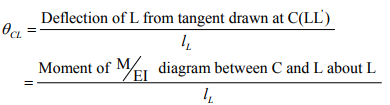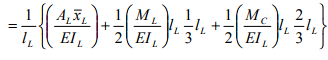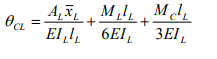(12.2)

Note that the actual moment diagram on span LC is broken into two parts (1) due to loads applied on span LC when it is considered as a simply supported beam and, (2) due to support moments. In the above equation AL and AR denote respectively area of the bending moment diagrams due to applied loads on left and right supports. xL and xR denote their respective C.G.(center of gravity) distances from the left and right support respectively. Similarly,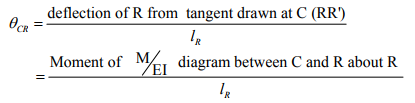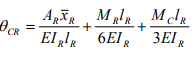Substituting the values of θCL and θCR in the compatibility equation                              (12.1),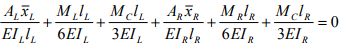(12.4)

which could be simplified to,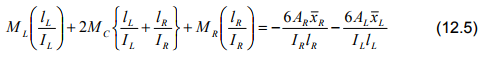The above equation (12.5) is known as the three-moment equation. It relates three support moments ML, MC and MR with the applied loading on two adjacent spans. If in a span there are more than one type of loading (for example, uniformly distributed load and a concentrated load) then it is simpler to calculate moment diagram separately for each of loading and then to obtain moment diagram.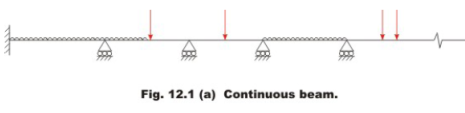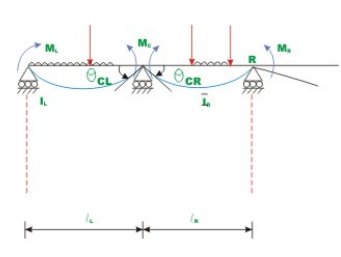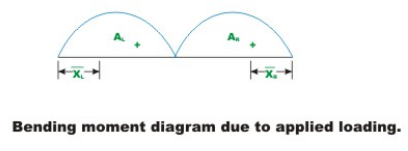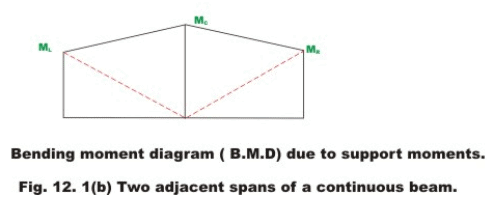Offer running on EduRev: Apply code STAYHOME200 to get INR 200 off on our premium plan EduRev Infinity!

## Structural Analysis

30 videos|122 docs|28 tests

,

,

,

,

,

,

,

,

,

,

,

,

,

,

,

,

,

,

,

,

,

;